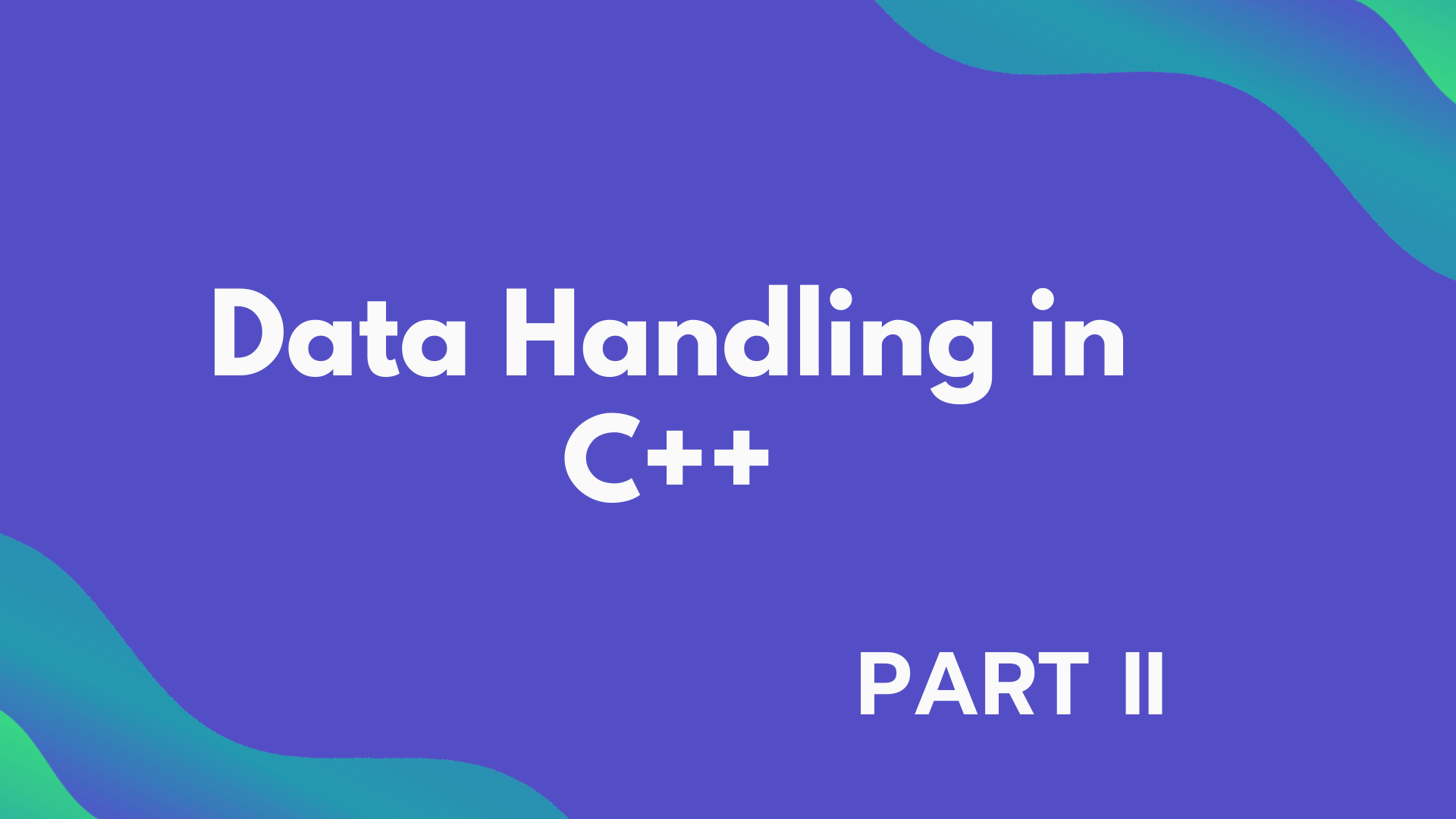# Types of Data Types (Data Handling Part 2)

## In this tutorial we will discuss the type of data type, there are five fundamental data types in C++: char, int, float, double, and void. All the other data types are based on these data types and those data types are known as derived data types.## Types of Data Type

### Fundamental Data Types

Those data types that are not composed of other data types are known are fundamental data types. We use variables to store data of specific data types, the variable is a named space to hold the value of a certain data type. Syntax to declare a variable for any fundamental data type is :

;

There are five fundamental data types in the C++ programming language :

1. int Data Type (for Integers)

1. It is used to represent integers, Integers are the whole number such as 5, 23, -256, etc.
2. It can not have a fractional value.
3. It can be negative or positive but only in this range -2147483648 to 2147483647.
4. Its size is usually 4 bytes.
``int num =5000;``

2. char Data Type (for characters)

1. char can store any character from the C++ basic character set.
2. Its size is 1 byte.
3. char in C++ is usually stored in a single quote ' '.
4. In C++ each character has an equivalent integer code which is known as ASCII code.
5. ASCII code stores number codes for 256 known characters: letter, digits, symbols, nano graphic characters, etc.
``char ch = 'A' ;``

3. float and double Data Type (for floating-point numbers)

1. A number having a fractional value is known as a floating-point number, for example, 2.893 is a floating-point number.
2. Floating-point variables are used to represent values like distance, area, temperature, etc.
3. The size of float data type is 4 bytes and the size of double data type is 8 bytes. `double` has two times the precision of float.
4. The range of float is 1.2E-38 to 3.4E+38 and the range of double is 2.3E-308 to 1.7E+308.
5. Floating point numbers can also be written as exponent notation. For example, 147.9101 can be written as 1.479101E02.

``````float pi = 3.14;
double area = 123.23243;``````

4. bool Data Type ( true or false)

1. The bool data type is used to represent "true" or "false".
2. The default numeric value of "true" is 1.
3. The default numeric value of  "false" is 0.
4. It is mostly used in conditional statements.
``````bool right = true;
bool wrong = false;``````

5. void Data Type ( for an empty set of values and non-returning functions)

1. The simple meaning of void is "null" or "no data".
2. The void data type is used to specify an empty set of values.
3. It is used as a return type of function which does not return any value.
4. We can not declare objects for a void data type.

### Derived Data Types

The data types which are derived using fundamental data types are known as Derived Data Types. for example Array, Pointers, References, Constants, etc.

Now, this the end of this tutorial we will learn about the Data Type Modifier in the next tutorial.0000000